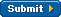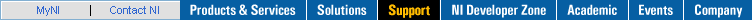# TFA Cone-Shaped Distribution VI

LabVIEW 2009 Advanced Signal Processing Toolkit Help

Edition Date: June 2009

Part Number: 372656A-01

»View Product Info

Owning Palette: Time Frequency Spectrogram VIs

Computes the Cone-Shaped Distribution (CSD) of signal. Wire data to the signal input to determine the polymorphic instance to use or manually select the instance.

Use the pull-down menu to select an instance of this VI.

 Select an instance TFA Cone-Shaped Distribution (Waveform)TFA Cone-Shaped Distribution (Real)TFA Cone-Shaped Distribution (Complex)

## TFA Cone-Shaped Distribution (Waveform)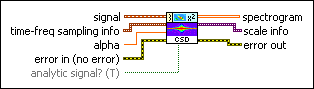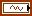signal specifies the input signal.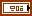time-frequency sampling info specifies the density to use to sample the signal in the joint time-frequency domain and defines the size of the resulting 2D time-frequency array.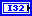time steps specifies the sampling period along the time axis in the joint time-frequency domain. The unit of time steps is in samples. The default is –1, which specifies that this VI adjusts time steps automatically so that no more than 512 rows exist in spectrogram. The number of rows in spectrogram equals the signal length divided by time steps.National Instruments recommends that you set time steps such that the number of rows in spectrogram does not exceed 512. If you specify a small value for time steps, this VI might return a large spectrogram, which requires a long computation time and more memory.If you need a small sampling period to observe more details and the signal length is large, divide the signal into smaller segments and compute the spectrogram for each segment. If the signal is oversampled, you also can downsample the signal. The scale info output contains the actual sampling period, in seconds, along the time axis.frequency bins specifies the number of bins along the frequency axis to sample the signal in the joint time-frequency domain. frequency bins must be a power of 2 and greater than 0. The scale info output contains the actual sampling period in hertz along the frequency axis.alpha specifies the relationship between resolution and cross-term interference. alpha must be greater than or equal to 0. Increasing alpha better suppresses cross-term interference but reduces the time-frequency resolution.error in (no error) describes error conditions that occur before this node runs. This input provides standard error in functionality.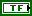analytic signal? specifies whether to convert the real input signal to the corresponding analytic signal. The default is TRUE. The analytic signal has no negative frequency components and has the same positive frequency components as the original signal. Converting the real input signal to the analytic signal can suppress the cross-term interference between the positive frequency components and the negative frequency components.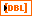spectrogram returns the quadratic time-frequency representation of the signal. Each row corresponds to the instantaneous power spectrum at a certain time. Use the TFA Spectrogram Indicator to display the spectrogram on an intensity graph. You can save the time-dependent 2D array to a text file for use in another software environment. The resulting text file contains only Z values and does not retain the time axis information or the frequency axis information. You can use the TFA Get Time and Freq Scale Info VI to compute the time scale information and the frequency scale information of the time-frequency representation.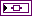scale info returns the time scale and the frequency scale information of the time-frequency representation, including the time offset, the time interval between every two contiguous rows, the frequency offset, and the frequency interval between every two contiguous columns of spectrogram. Use the TFA Get Time and Freq Scale Info VI to return detailed information about the time scale and the frequency scale.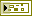error out contains error information. This output provides standard error out functionality.

## TFA Cone-Shaped Distribution (Real)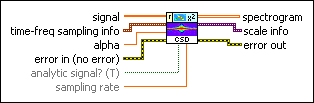signal specifies the input signal.time-frequency sampling info specifies the density to use to sample the signal in the joint time-frequency domain and defines the size of the resulting 2D time-frequency array.time steps specifies the sampling period along the time axis in the joint time-frequency domain. The unit of time steps is in samples. The default is –1, which specifies that this VI adjusts time steps automatically so that no more than 512 rows exist in spectrogram. The number of rows in spectrogram equals the signal length divided by time steps.National Instruments recommends that you set time steps such that the number of rows in spectrogram does not exceed 512. If you specify a small value for time steps, this VI might return a large spectrogram, which requires a long computation time and more memory.If you need a small sampling period to observe more details and the signal length is large, divide the signal into smaller segments and compute the spectrogram for each segment. If the signal is oversampled, you also can downsample the signal. The scale info output contains the actual sampling period, in seconds, along the time axis.frequency bins specifies the number of bins along the frequency axis to sample the signal in the joint time-frequency domain. frequency bins must be a power of 2 and greater than 0. The scale info output contains the actual sampling period in hertz along the frequency axis.alpha specifies the relationship between resolution and cross-term interference. alpha must be greater than or equal to 0. Increasing alpha better suppresses cross-term interference but reduces the time-frequency resolution.error in (no error) describes error conditions that occur before this node runs. This input provides standard error in functionality.analytic signal? specifies whether to convert the real input signal to the corresponding analytic signal. The default is TRUE. The analytic signal has no negative frequency components and has the same positive frequency components as the original signal. Converting the real input signal to the analytic signal can suppress the cross-term interference between the positive frequency components and the negative frequency components.sampling rate specifies the sampling rate of signal in hertz. sampling rate must be greater than 0, or the VI sets sampling rate to 1 automatically. The default is 1.spectrogram returns the quadratic time-frequency representation of the signal. Each row corresponds to the instantaneous power spectrum at a certain time. Use the TFA Spectrogram Indicator to display the spectrogram on an intensity graph. You can save the time-dependent 2D array to a text file for use in another software environment. The resulting text file contains only Z values and does not retain the time axis information or the frequency axis information. You can use the TFA Get Time and Freq Scale Info VI to compute the time scale information and the frequency scale information of the time-frequency representation.scale info returns the time scale and the frequency scale information of the time-frequency representation, including the time offset, the time interval between every two contiguous rows, the frequency offset, and the frequency interval between every two contiguous columns of spectrogram. Use the TFA Get Time and Freq Scale Info VI to return detailed information about the time scale and the frequency scale.error out contains error information. This output provides standard error out functionality.

## TFA Cone-Shaped Distribution (Complex)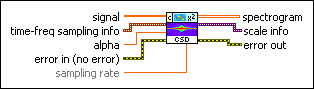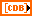signal specifies the input signal.time-frequency sampling info specifies the density to use to sample the signal in the joint time-frequency domain and defines the size of the resulting 2D time-frequency array.time steps specifies the sampling period along the time axis in the joint time-frequency domain. The unit of time steps is in samples. The default is –1, which specifies that this VI adjusts time steps automatically so that no more than 512 rows exist in spectrogram. The number of rows in spectrogram equals the signal length divided by time steps.National Instruments recommends that you set time steps such that the number of rows in spectrogram does not exceed 512. If you specify a small value for time steps, this VI might return a large spectrogram, which requires a long computation time and more memory.If you need a small sampling period to observe more details and the signal length is large, divide the signal into smaller segments and compute the spectrogram for each segment. If the signal is oversampled, you also can downsample the signal. The scale info output contains the actual sampling period, in seconds, along the time axis.frequency bins specifies the number of bins along the frequency axis to sample the signal in the joint time-frequency domain. frequency bins must be a power of 2 and greater than 0. The scale info output contains the actual sampling period in hertz along the frequency axis.alpha specifies the relationship between resolution and cross-term interference. alpha must be greater than or equal to 0. Increasing alpha better suppresses cross-term interference but reduces the time-frequency resolution.error in (no error) describes error conditions that occur before this node runs. This input provides standard error in functionality.sampling rate specifies the sampling rate of signal in hertz. sampling rate must be greater than 0, or the VI sets sampling rate to 1 automatically. The default is 1.spectrogram returns the quadratic time-frequency representation of the signal. Each row corresponds to the instantaneous power spectrum at a certain time. Use the TFA Spectrogram Indicator to display the spectrogram on an intensity graph. You can save the time-dependent 2D array to a text file for use in another software environment. The resulting text file contains only Z values and does not retain the time axis information or the frequency axis information. You can use the TFA Get Time and Freq Scale Info VI to compute the time scale information and the frequency scale information of the time-frequency representation.scale info returns the time scale and the frequency scale information of the time-frequency representation, including the time offset, the time interval between every two contiguous rows, the frequency offset, and the frequency interval between every two contiguous columns of spectrogram. Use the TFA Get Time and Freq Scale Info VI to return detailed information about the time scale and the frequency scale.error out contains error information. This output provides standard error out functionality.

## TFA Cone-Shaped Distribution Details

CSD is a type of Cohen's class distribution and is the smooth version of the Wigner-Ville Distribution (WVD). CSD has better readability than WVD but worse time-frequency resolution, because CSD suppresses the cross-term interference between two signal components that have a large difference in central time or central frequency. However, CSD does not suppress the cross-term interference between two signal components that have the same central time. Therefore, for large values of the signal length, this VI requires a long computation time and more memory. National Instruments recommends that you limit signal to 15,000 samples.

Your Feedback!Poor  |  ExcellentYes No
 Document Quality?Add Comments 1 2 3 4 5